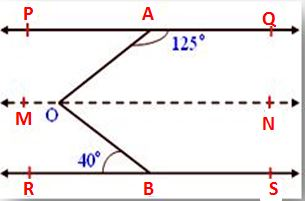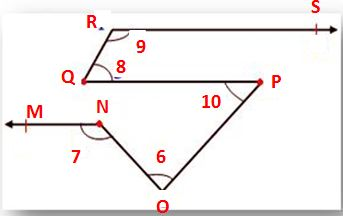# Parallel and Transversal Lines | Properties of Parallel Lines cut by Transversal

Learn how a pair of angles formed between Parallel and Transversal Lines. When two parallel lines intersect by a Transversal Line, they form different angles. Those angles are given with different names based on their location. Therefore, check what are the angles formed and how to find them, and what names are given to them depending on their location. You can also get all concepts and examples available on Lines and Angles for free on our website.

When the transversal intersects two parallel lines

• Pairs of alternate angles are equal
• Pairs of corresponding angles are equal.
• Interior angles on the same side of the transversal are supplementary.

## Parallel Lines Cut by a Transversal Problems

1. In adjoining figure l ∥ m is cut by the transversal t. If ∠5 = 70, find the measure of ∠7, ∠9, ∠10.Solution:
Given that ∠5 = 70° ∠5 = ∠7 (Vertically opposite angles)
Therefore, ∠7 = 70°
Now, ∠5 = ∠9 (Corresponding angles)
Therefore, ∠9 = 70°
Also, ∠7 + ∠10 = 180° (Co-interior angles)
70° + ∠10 = 180°

Therefore, ∠10 = 180° – 70° = 110°

2. In the given figure PQ ∥ RS, ∠QAO = 125°, ∠RBO = 40°. Find the measure of ∠AOB.

Solution:Draw a line AB parallel to PQ and RS passing through O such that PQ ∥ MN and RS ∥ MN
∠QAO + ∠NOA = 180° (Co-interior angles)
Therefore, 125° + ∠NOA = 180°
Therefore, ∠NOA = 180° – 125° = 55°
Also, ∠RBO = ∠NOB (Alternate angles)
Given ∠RBO = 40°
Therefore, ∠NOB = 40°
Then ∠AOB = ∠AON + ∠BON
= 55° + 40° = 95°

3. In the given figure NM ∥ PO ∥ SR and NS ⊥ NM. Also, ∠MNS = 90°. Find the values of ∠a, ∠b and ∠c.

Solution:b + 45° = 180°
Therefore, ∠b = 180° – 45° (Co-interior angles) = 135°
∠b =∠a (Corresponding angles)
Therefore, ∠a = 135°
Also, 90° + ∠c + 45° = 180°
Therefore, 135° + ∠c = 180°
Therefore, ∠c = 180° – 135° = 45°

The final answer is ∠a = 135°, ∠b = 135°, ∠c = 45°

4. In the given figure, MN ∥ QP, QP ∥ RS, QR ∥ OP. Also, ∠6 = 60°, ∠8 = 55°, then find ∠7, ∠9, ∠10.

Solution:Since, QR ∥ OP cut by transversal QP
Therefore, ∠8 = ∠10 we know, ∠8 = 55°
Therefore, ∠10 = 55°
Also, QP ∥ XY cut by transversal OP
Therefore, ∠10 = ∠x we know ∠10 = 55°
Therefore,∠x = 55°
Also, ∠x + ∠1 + ∠y = 180°
55° + 60° + ∠y = 180°
115° + ∠y = 180°
∠y = 180° – 115°
Therefore, ∠y = 65°
Now, ∠y + ∠2 = 1800 (Co-interior angles)65° + ∠7 = 180°
∠7 = 180° – 65°
∠7 = 115°
Since, QP ∥ RS cut by transversal QR
Therefore, ∠8 + ∠9 = 180°
55° + ∠9 = 180°
Therefore, ∠9 = 180° – 55° = 125°

The final answer is ∠7 = 115°, ∠9 = 125°, ∠10 = 55°.

5. In the given figure AB ∥ EF. Also, m : n = 4 : 5 find.Solution:
Let the common ratio be x
Then m = 4x and n = 5x
Also, ∠n = ∠o (Alternate interior angles)
Since, n = 5x
Therefore, ∠o = 5x [CD ∥ EF cut by transversal t]
Now, ∠o = ∠l (Corresponding angles)
Since, ∠o = 5x
Therefore, ∠l = 5x [AB ∥ CD cut by transversal t]
∠l + ∠m = 180° (Co-interior angles)
5x + 4x = 1800
9x = 180°
x = 180/9
x = 20
Since, m = 4x
Therefore, m = 4 × 20
m = 80°
n = 5x
Therefore, n = 5 × 20
n = 100°
l = 5x
Therefore, l = 5 × 20
l = 100°

Therefore, ∠l = 100°, ∠m = 80°, ∠n = 100°